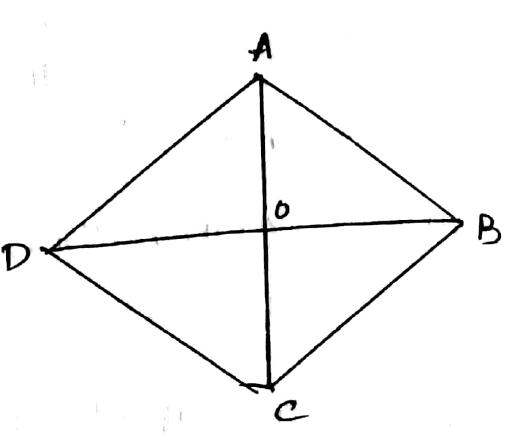Question

Prove that the rhombus with equal diagonals is a square.

Solution$$ABCD$$ is a rhombus, in which diagonals AC and BD are equal.We know that diagonals of rhombus bisect each other.As $$AC=BD$$$$\therefore AO=BO=CO=DO$$In $$\triangle AOB,$$$$\Rightarrow AO=OB$$ and $$\angle AOD=90°$$$$\therefore \angle OAB=\angle OBA=\dfrac{90^o}{2}=45°$$Similarly in $$\triangle AOD,$$$$\Rightarrow \angle OAD=\angle ODA=45°$$$$\therefore \angle A=\angle OAB+\angle OAD=45^o+45^o=90°$$Similarly, $$\Rightarrow \angle B=\angle C=\angle D=90°$$here, $$AB=BC=CD=DA$$$$\therefore$$ Quadrilateral ABCD is a square. Mathematics

Suggest Corrections0Similar questions
View MorePeople also searched for
View More